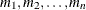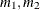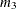# The GLIMMIX Procedure

### PARMS Statement

Subsections:
• PARMS <(value-list)> …</ options>;

The PARMS statement specifies initial values for the covariance or scale parameters, or it requests a grid search over several values of these parameters in generalized linear mixed models.

The value-list specification can take any of several forms:

m

a single valueseveral values

m to n

a sequence where m equals the starting value, n equals the ending value, and the increment equals 1

m to n by i

a sequence where m equals the starting value, n equals the ending value, and the increment equals itomixed values and sequences

#### Using the PARMS Statement with a GLM

If you are fitting a GLM or a GLM with overdispersion, the scale parameters are listed at the end of the "Parameter Estimates" table in the same order as value-list. If you specify more than one set of initial values, PROC GLIMMIX uses only the first value listed for each parameter. Grid searches by using scale parameters are not possible for these models, because the fixed effects are part of the optimization.

#### Using the PARMS Statement with a GLMM

If you are fitting a GLMM, the value-list corresponds to the parameters as listed in the "Covariance Parameter Estimates" table. Note that this order can change depending on whether a residual variance is profiled or not; see the NOPROFILE option in the PROC GLIMMIX statement.

If you specify more than one set of initial values, PROC GLIMMIX performs a grid search of the objective function surface and uses the best point on the grid for subsequent analysis. Specifying a large number of grid points can result in long computing times.

#### Options in the PARMS Statement

You can specify the following options in the PARMS statement after a slash (/).

HOLD=value-list

specifies which parameter values PROC GLIMMIX should hold equal to the specified values. For example, the following statement constrains the first and third covariance parameters to equal 5 and 2, respectively:

parms (5) (3) (2) (3) / hold=1,3;


Covariance or scale parameters that are held fixed with the HOLD= option are treated as constrained parameters in the optimization. This is different from evaluating the objective function, gradient, and Hessian matrix at known values of the covariance parameters. A constrained parameter introduces a singularity in the optimization process. The covariance matrix of the covariance parameters (see the ASYCOV option of the PROC GLIMMIX statement) is then based on the projected Hessian matrix. As a consequence, the variance of parameters subjected to a HOLD= is zero. Such parameters do not contribute to the computation of denominator degrees of freedom with the DDFM= KENWARDROGER and DDFM= SATTERTHWAITE methods, for example. If you want to treat the covariance parameters as known, without imposing constraints on the optimization, you should use the NOITER option.

When you place a hold on all parameters (or when you specify the NOITER) option in a GLMM, you might notice that PROC GLIMMIX continues to produce an iteration history. Unless your model is a linear mixed model, several recomputations of the pseudo-response might be required in linearization-based methods to achieve agreement between the pseudo-data and the covariance matrix. In other words, the GLIMMIX procedure continues to update the fixed-effects estimates (and random-effects solutions) until convergence is achieved.

In certain models, placing a hold on covariance parameters implies that the procedure processes the parameters in the same order as if the NOPROFILE were in effect. This can change the order of the covariance parameters when you place a hold on one or more parameters. Models that are subject to this reordering are those with R-side covariance structures whose scale parameter could be profiled. This includes the TYPE=CS , TYPE=SP , TYPE=AR(1) , TYPE=TOEP , and TYPE=ARMA(1,1) covariance structures.

LOWERB=value-list

enables you to specify lower boundary constraints for the covariance or scale parameters. The value-list specification is a list of numbers or missing values (.) separated by commas. You must list the numbers in the same order that PROC GLIMMIX uses for the value-list in the PARMS statement, and each number corresponds to the lower boundary constraint. A missing value instructs PROC GLIMMIX to use its default constraint, and if you do not specify numbers for all of the covariance parameters, PROC GLIMMIX assumes that the remaining ones are missing.

This option is useful, for example, when you want to constrain thematrix to be positive definite in order to avoid the more computationally intensive algorithms required whenbecomes singular. The corresponding statements for a random coefficients model are as follows:

proc glimmix;
class person;
model y = time;
random int time / type=chol sub=person;
parms / lowerb=1e-4,.,1e-4;
run;


Here, the TYPE=CHOL structure is used in order to specify a Cholesky root parameterization for theunstructured blocks in. This parameterization ensures that thematrix is nonnegative definite, and the PARMS statement then ensures that it is positive definite by constraining the two diagonal terms to be greater than or equal to 1E–4.

NOBOUND

requests the removal of boundary constraints on covariance and scale parameters in mixed models. For example, variance components have a default lower boundary constraint of 0, and the NOBOUND option allows their estimates to be negative. See the NOBOUND option in the PROC GLIMMIX statement for further details.

NOITER

requests that no optimization of the covariance parameters be performed. This option has no effect in generalized linear models.

If you specify the NOITER option, PROC GLIMMIX uses the values for the covariance parameters given in the PARMS statement to perform statistical inferences. Note that the NOITER option is not equivalent to specifying a HOLD= value for all covariance parameters. If you use the NOITER option, covariance parameters are not constrained in the optimization. This prevents singularities that might otherwise occur in the optimization process.

If a residual variance is profiled, the parameter estimates can change from the initial values you provide as the residual variance is recomputed. To prevent an update of the residual variance, combine the NOITER option with the NOPROFILE option in the PROC GLIMMIX statements, as in the following code:

proc glimmix noprofile;
class A B C rep mp sp;
model y = A | B | C;
random rep mp sp;
parms (180) (200) (170) (1000) / noiter;
run;


When you specify the NOITER option in a model where parameters are estimated by pseudo-likelihood techniques, you might notice that the GLIMMIX procedure continues to produce an iteration history. Unless your model is a linear mixed model, several recomputations of the pseudo-response might be required in linearization-based methods to achieve agreement between the pseudo-data and the covariance matrix. In other words, the GLIMMIX procedure continues to update the profiled fixed-effects estimates (and random-effects solutions) until convergence is achieved. To prevent these updates, use the MAXLMMUPDATE= option in the PROC GLIMMIX statement. Specifying the NOITER option in the PARMS statement of a GLMM with pseudo-likelihood estimation has the same effect as choosing TECHNIQUE=NONE in the NLOPTIONS statement.

If you want to base initial fixed-effects estimates on the results of fitting a generalized linear model, then you can combine the NOITER option with the TECHNIQUE= option. For example, the following statements determine the starting values for the fixed effects by fitting a logistic model (without random effects) with the Newton-Raphson algorithm:

proc glimmix startglm inititer=10;
class clinic A;
model y/n = A / link=logit dist=binomial;
random clinic;
parms (0.4) / noiter;
nloptions technique=newrap;
run;


The initial GLM fit stops at convergence or after at most 10 iterations, whichever comes first. The pseudo-data for the linearized GLMM is computed from the GLM estimates. The variance of the Clinic random effect is held constant at 0.4 during subsequent iterations that update the fixed effects only.

If you also want to combine the GLM fixed-effects estimates with known and fixed covariance parameter values without updating the fixed effects, you can add the MAXLMMUPDATE =0 option:

proc glimmix startglm inititer=10 maxlmmupdate=0;
class clinic A;
model y/n = A / link=logit dist=binomial;
random clinic;
parms (0.4) / noiter;
nloptions technique=newrap;
run;


In a GLMM with parameter estimation by METHOD= LAPLACE or METHOD= QUAD the NOITER option also leads to an iteration history, since the fixed-effects estimates are part of the optimization and the PARMS statement places restrictions on only the covariance parameters.

Finally, the NOITER option can be useful if you want to obtain minimum variance quadratic unbiased estimates (with 0 priors), also known as MIVQUE0 estimates (Goodnight 1978a). Because MIVQUE0 estimates are starting values for covariance parameters—unless you provide (value-list) in the PARMS statement—the following statements produce MIVQUE0 mixed model estimates:

proc glimmix noprofile;
class A B;
model y = A;
random int / subject=B;
parms / noiter;
run;

PARMSDATA=SAS-data-set
PDATA=SAS-data-set

reads in covariance parameter values from a SAS data set. The data set should contain the numerical variable ESTIMATE or the numerical variables Covp1Covpq, where q denotes the number of covariance parameters.

If the PARMSDATA= data set contains multiple sets of covariance parameters, the GLIMMIX procedure evaluates the initial objective function for each set and commences the optimization step by using the set with the lowest function value as the starting values. For example, the following SAS statements request that the objective function be evaluated for three sets of initial values:

data data_covp;
input covp1-covp4;
datalines;
180 200 170 1000
170 190 160  900
160 180 150  800
;
proc glimmix;
class A B C rep mainEU smallEU;
model yield = A|B|C;
random rep mainEU smallEU;
parms / pdata=data_covp;
run;


Each set comprises four covariance parameters.

The order of the observations in a data set with the numerical variable Estimate corresponds to the order of the covariance parameters in the "Covariance Parameter Estimates" table. In a GLM, the PARMSDATA= option can be used to set the starting value for the exponential family scale parameter. A grid search is not conducted for GLMs if you specify multiple values.

The PARMSDATA= data set must not contain missing values.

If the GLIMMIX procedure is processing the input data set in BY groups, you can add the BY variables to the PARMSDATA= data set. If this data set is sorted by the BY variables, the GLIMMIX procedure matches the covariance parameter values to the current BY group. If the PARMSDATA= data set does not contain all BY variables, the data set is processed in its entirety for every BY group and a message is written to the log. This enables you to provide a single set of starting values across BY groups, as in the following statements:

data data_covp;
input covp1-covp4;
datalines;
180 200 170 1000
;

proc glimmix;
class A B C rep mainEU smallEU;
model yield = A|B|C;
random rep mainEU smallEU;
parms / pdata=data_covp;
by year;
run;


The same set of starting values is used for each value of the year variable.

UPPERB=value-list

enables you to specify upper boundary constraints on the covariance parameters. The value-list specification is a list of numbers or missing values (.) separated by commas. You must list the numbers in the same order that PROC GLIMMIX uses for the value-list in the PARMS statement, and each number corresponds to the upper boundary constraint. A missing value instructs PROC GLIMMIX to use its default constraint. If you do not specify numbers for all of the covariance parameters, PROC GLIMMIX assumes that the remaining ones are missing.